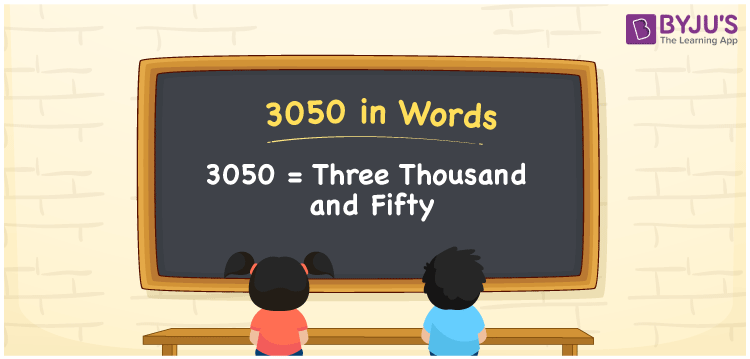# 3050 in Words

3050 in words can be written as Three Thousand and Fifty. Students will be able to learn the conversion of 3050 in words which will help them understand the applications of numbers in our daily lives. If you buy a watch for Rs. 3050, then you can say that “I have bought a watch for Three Thousand and Fifty Rupees”. The  number 3050 can be written in words using the English alphabet. The numbers in words can be grasped easily by the students using the resources given at BYJU’S. 3050 in English can be read as “Three Thousand and Fifty”.

 3050 in words Three Thousand and Fifty Three Thousand and Fifty in Numbers 3050

## 3050 in English Words## How to Write 3050 in Words?

Students will learn about the conversion of 3050 into words from place value charts. The number 3050 has four digits. For 3050, the place value chart is prepared in a table form to help students understand it effectively.

 Thousands Hundreds Tens Ones 3 0 5 0

3050 in expanded form is explained in brief here:

3 × Thousand + 0 × Hundred + 5 × Ten + 0 × One

= 3 × 1000  + 0 × 100 + 5 × 10 + 0 × 1

= 3000 + 50

= 3050

= Three Thousand and Fifty

Therefore, 3050 in words is written as Three Thousand and Fifty.

3050 is a natural number that precedes 3051 and succeeds 3049.

3050 in words – Three Thousand and Fifty

Is 3050 an odd number? – No

Is 3050 an even number? – Yes

Is 3050 a perfect square number? – No

Is 3050 a perfect cube number? – No

Is 3050 a prime number? – No

Is 3050 a composite number? – Yes

## Frequently Asked Questions on 3050 in Words

Q1

### How do you write 3050 in words?

3050 can be written as “Three Thousand and Fifty” in words.
Q2

### Is 3050 an even number?

3050 is an even number because it is divisible by 2.
3050/2 = 1525
Q3

### How can Three Thousand and Fifty be written in numbers?

Three Thousand and Fifty can be written in numbers as 3050.